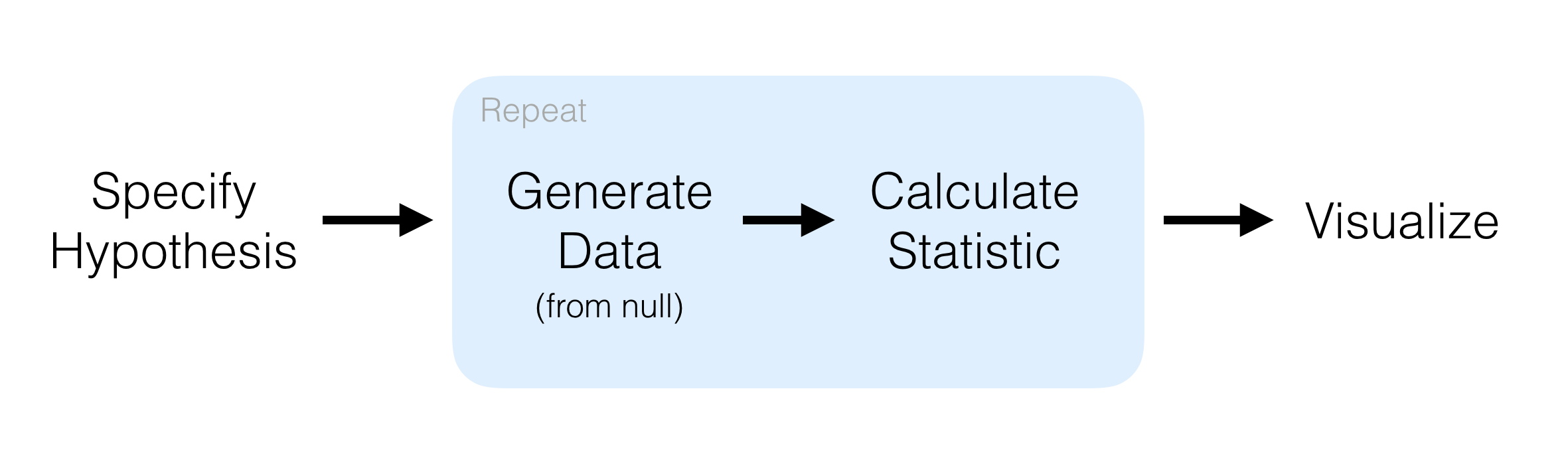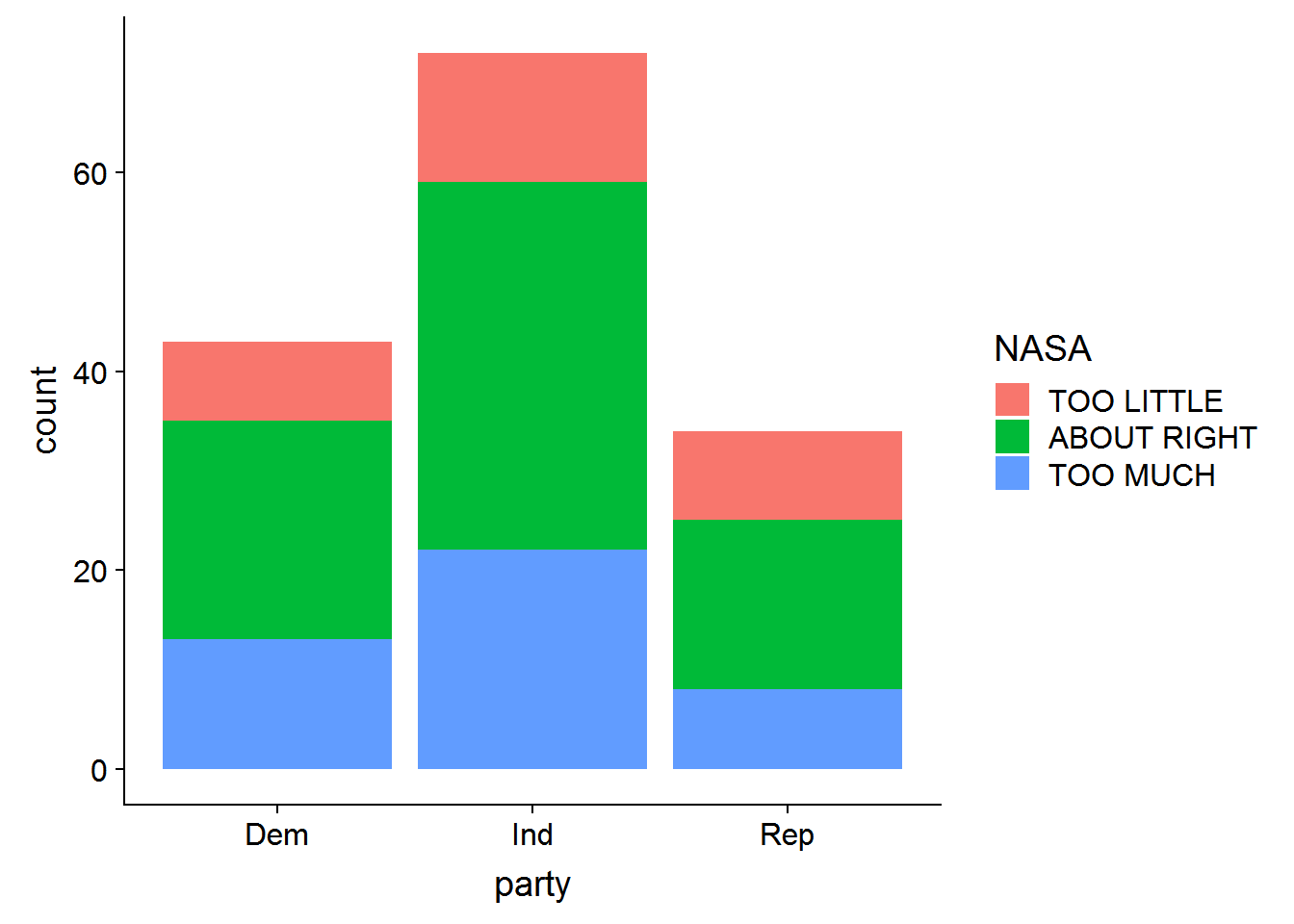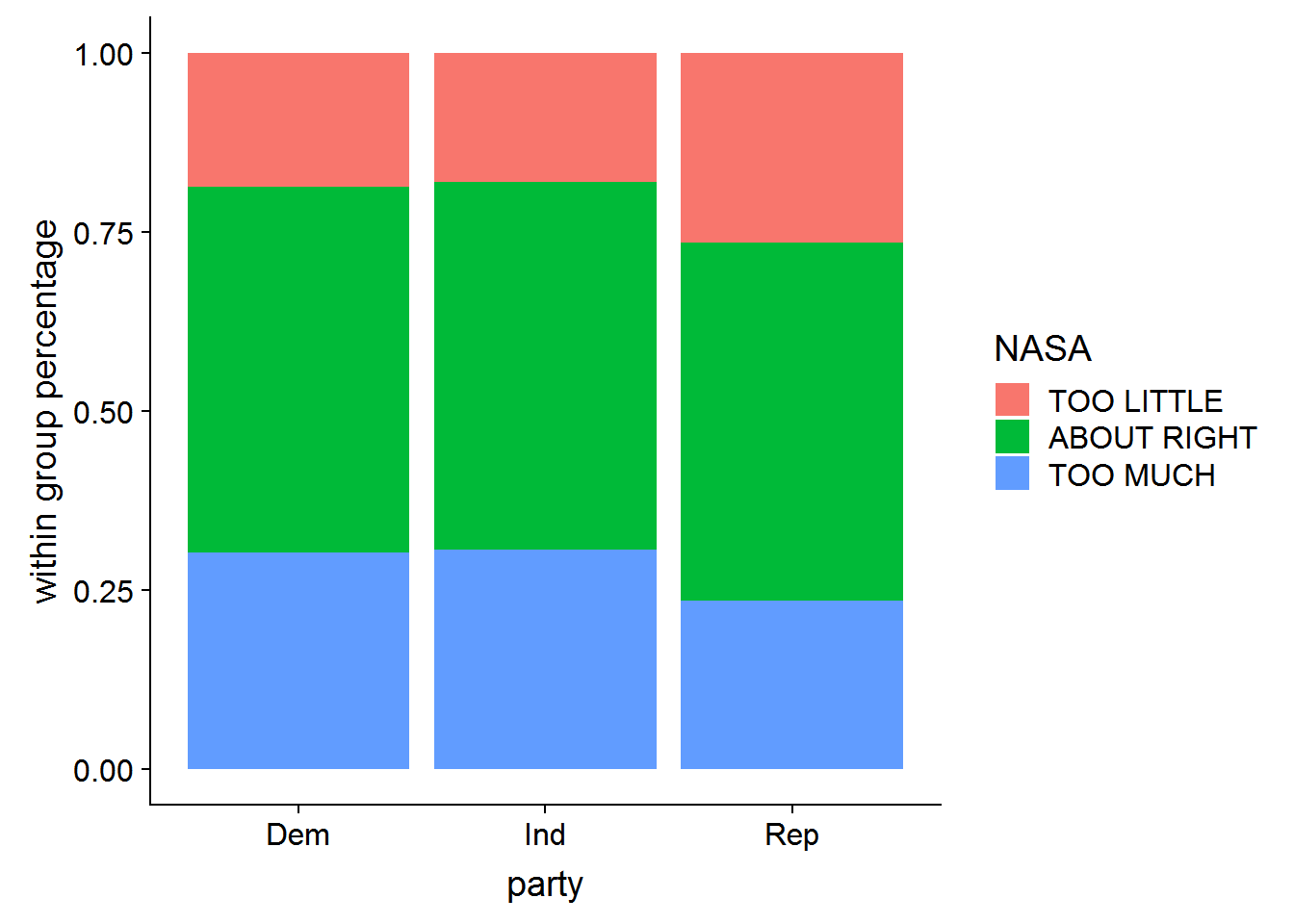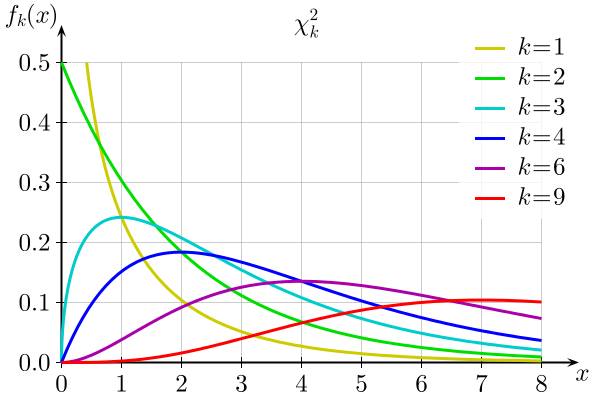This blog post is my attempt to process and expand on an excellent talk delivered by Andrew Bray at rstudio::conf2018. The slides (PDF) from Andrew’s presentation can be found at this github link.

From Infer’s homepage:

The objective of this package is to perform statistical inference using an expressive statistical grammar that coheres with the tidyverse design framework.Let’s see how that’s done. First we install `infer` and load it into our environment.

``````# install.packages("infer")
library(infer)
library(cowplot) # for nice plots``````

Infer is supposed to play well within the tidyverse, so we load that too, along with some data from the General Social Survey. In this dataset, we see the diversity of information collected.

``````library(tidyverse)
names(gss)``````
``````##   "id"       "year"     "age"      "class"    "degree"   "sex"
##   "marital"  "race"     "region"   "partyid"  "happy"    "relig"
##  "cappun"   "finalter" "natspac"  "natarms"  "conclerg" "confed"
##  "conpress" "conjudge" "consci"   "conlegis" "zodiac"   "oversamp"
##  "postlife" "party"    "space"    "NASA"``````

## Question: Is funding for space exploration (NASA) a partisan issue?

Let’s start by looking at responses to the question: “Do you think funding for space exploration is too little, about right, or too much?”

``gss %>% select(party, NASA)``
``````## # A tibble: 149 x 2
##    party NASA
##    <fct> <fct>
##  1 Ind   TOO LITTLE
##  4 Ind   TOO LITTLE
##  5 Ind   TOO MUCH
##  6 Ind   TOO LITTLE
##  9 Dem   TOO LITTLE
## 10 Ind   TOO LITTLE
## # ... with 139 more rows``````

We see that people can either select too little, about right, or too much. We can visualize this pretty easily:

``````gss %>%
select(party, NASA) %>%
ggplot(aes(x=party, fill = NASA)) +
geom_bar()``````Count data can be misleading when we’re looking for trends between categorical variables within groups, so let’s normalize within-group-percentages with a `position = "fill"` argument in `geom_bar()`.

``````gss %>%
select(party, NASA) %>%
ggplot(aes(x=party, fill = NASA)) +
geom_bar(position = "fill") +
ylab("within group percentage")``````It doesn’t look like there’s much of a difference in how Democrats, Independents, and Republicans support space exploration, but let’s now drill down into this with some hypothesis testing, comparing Base R and `infer`.

What we essentially have is a contingency table of party affiliation and attitude towards space exploration, and we want to see if there’s a relationship between these variables. The Chi Squared Test of independence is used to determine if a significant relationship exists between two categorical variables, so we will use this test.

• Null hypothesis: There is no realationship between party (Democrat, Independent, Republican) and attitude towards space exploration (too little, about right, too much).
• Alternative hypothesis: There is a relationship between party and attitude towards space exploration.

Before we perform a Chi-squared test, in base R and `infer`, let’s quickly break down what a Chi-squared test is all about.

We have our data (party and attitude towards space exploration), we assume:

• Assumption #1: Variable are categorical. There are 3 types of categorical data:
• Dichotomous: 2 groups (eg - Male and Female)
• Nominal: 3 or more categorical groups (eg - undergrad, professor, graduate student, postdoctoral scholar)
• Ordinal: ordered groups (eg - Pain Level 1, Pain Level 2, Pain Level 3, …)
• Assumption #2: Observations are independent of one another (eg - no relationship between any of the cases).
• Assumption #3: Categorical variable groupings must be mutually exclusive. In other words, we can’t have one participant as “Democrat” and “Independent”.
• Assumption #4: There must be at least 5 expected frequencies in each group of your categorical variable (only important for the analytical solution ).

We meet those assumptions, so let’s power ahead!

There are two main ways to solve this problem:

1. Analytically (mathmematically)
2. Programatically

## Analytical SolutionChi-squared probability density function (Wikipedia). k = degrees of freedom

We assume the expected values follow a Chi-squared distribution, with a probability density function that depends on the degrees of freedom. The plot above shows how the distribution varies with the degrees of freedom (k). On the x axis is the Chi-squared statistic, which we can calculate in R. We could then see where it falls in the distribution, and observe the probability of arriving at that combination of variables, or a more extreme example. As our Chi-squared test statistic increases, we move further along the x axis to the right. There is less area under the curve to the right, and our p-value (the area under the curve to the right of the observed statistic) decreases.

Generally speaking, a larger Chi-squared statistic suggests stronger evidence for rejecting our null hhypothesis. If we observe a p-value <= .05, we would reject our null hypothesis.

What would it mean to accept our alternative hypothesis?

In the case of our example, if we we lived in a completely random universe, less than 5% of the time we would arrive at the particular combination of party and attitude towards space exporlation we observe in our data. In other words, the relationship between party and attitude towards space exploration we see in our data is significant.

But is there actually a significant relationship between these variables? We will calculate a Chi-squared statistic for our data to find out.

Base R’s `stats` package has a function that takes two vectors of data, and returns a Chi-squared test statistic, degrees of freedom, and p-value. Let’s save this observed Chi statistic for later use.

``chisq.test(gss\$party, gss\$NASA) ``
``````##
##  Pearson's Chi-squared test
##
## data:  gss\$party and gss\$NASA
## X-squared = 1.3261, df = 4, p-value = 0.8569``````
``observed_stat <- chisq.test(gss\$party, gss\$NASA)\$stat``

We might be tempted to look at this and say, there’s a high p-value. No significant relationship exists. Job done. This is what we expected looking at the bar plots earlier. But let’s go a step further with `infer`.

## Programatic Solution

Another way to test if there is a significant relationship in our data is to take a programatic approach.

In his talk, Andrew Bray says:

If we live in world where variables are totally unrelated, the ties between variables are arbitrary, so they might just as well have been shuffled.

What would that random world look like? Let’s take one of the columns of our data_frame and scramble it.

``````gss %>% select(party, NASA) %>%
mutate(permutation_1 = sample(NASA),
permutation_2 = sample(NASA))``````
``````## # A tibble: 149 x 4
##    party NASA        permutation_1 permutation_2
##    <fct> <fct>       <fct>         <fct>